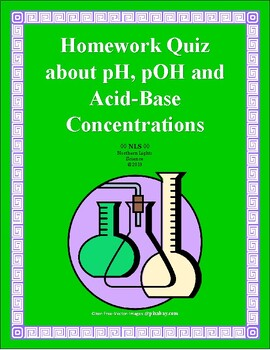# Homework Quiz or Worksheet on pH, pOH, [H+], [OH-] Acid-Base Conversion ProblemsSubject
Resource Type
Product Rating
File Type

Word Document File

Be sure that you have an application to open this file type before downloading and/or purchasing.

23 KB|2 pages
Share
Product Description

This one-page homework quiz checks the students’ understanding of pH and pOH, and their relationships to [H+] and [OH-]. The students’ objective is to complete all seven problems – calculate pH from a molar solution of an acid [HCl], calculate the hydrogen ion concentration [H+] if given the pH, calculate the pOH if given the hydroxide ion concentration [OH-], calculate the hydroxide ion concentration [OH-] if given the pOH, calculate the pH if given the concentration of the strong base [OH-], calculate the concentration of the base [OH-] if given the concentration of the acid [H+], and calculate the concentration of the acid [H+] from a pOH value.

I included an equation key in the upper left corner of the student sheet that displays six key equations. I also added a text box at the bottom of the quiz, reminding them of the significant figure rule for standard numbered values like molarity versus the unique rule where only the numbers after the decimal are significant in pH and pOH.

I provided a one-page answer key that shows the work with answers in colored font. This quiz is editable. This activity could be renamed and used as a worksheet instead. On a side note, the problems are more spaced out than what the thumbnails show.

I offer dozens of quality field-tested, innovative, practical, and user-friendly science products for several fields of science - biology, chemistry, field ecology, physical science, earth science, and human anatomy and physiology.

I began launching my curriculum through TpT in 2018. I have over twenty years of teaching and curriculum writing experience as the science department head. I joined TpT with seventeen years of publishing experience through several international science supply corporations.

Total Pages
2 pages